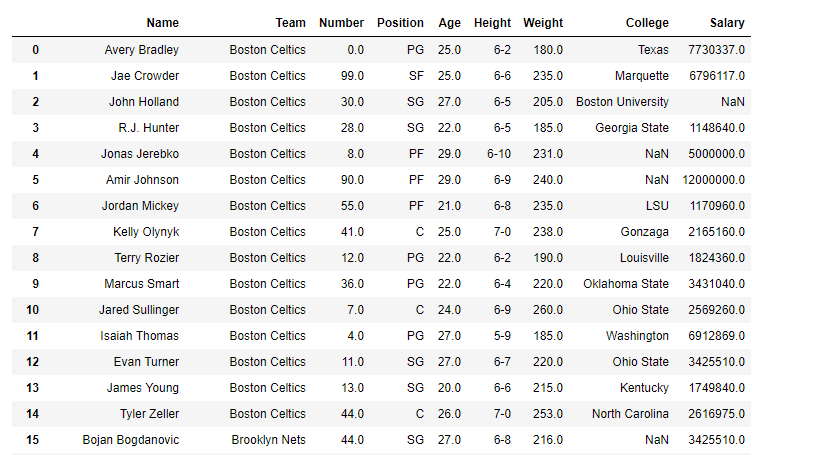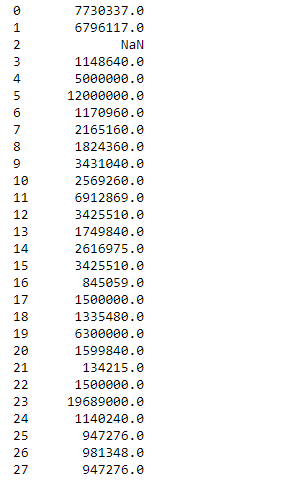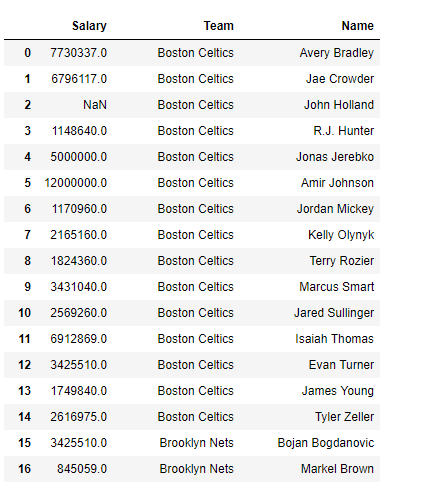Open In App

# Python | Pandas dataframe.get()

Python is a great language for doing data analysis, primarily because of the fantastic ecosystem of data-centric python packages. Pandas is one of those packages and makes importing and analyzing data much easier.

Pandas` dataframe.get()` function is used to get item from object for given key. The key could be one or more than one dataframe column. It returns default value if not found.

Syntax: DataFrame.get(key, default=None)

Parameters :
key : object

Returns : value : type of items contained in object

Example #1: Use `get()` function to extract a column out of the dataframe

 `# importing pandas as pd``import` `pandas as pd`` ` `# Creating the dataframe ``df ``=` `pd.read_csv(``"nba.csv"``)`` ` `# Print the dataframe``df`

Output :Now apply the `get()` function. We are going to extract the “Salary” column from the dataframe.

 `# applying get() function ``df.get(``"Salary"``)`

Output :Notice, the output is not a dataframe but a pandas series object.

Example #2: Use `get()` function to extract multiple columns at a time in random order

 `# importing pandas as pd``import` `pandas as pd`` ` `# Creating the dataframe ``df ``=` `pd.read_csv(``"nba.csv"``)`` ` `df.get([``"Salary"``, ``"Team"``, ``"Name"``])`

Output :A dataframe is returned as output. The ordering of the columns is not according to the actual dataframe but it follows the ordering that we provided to it in the function input.